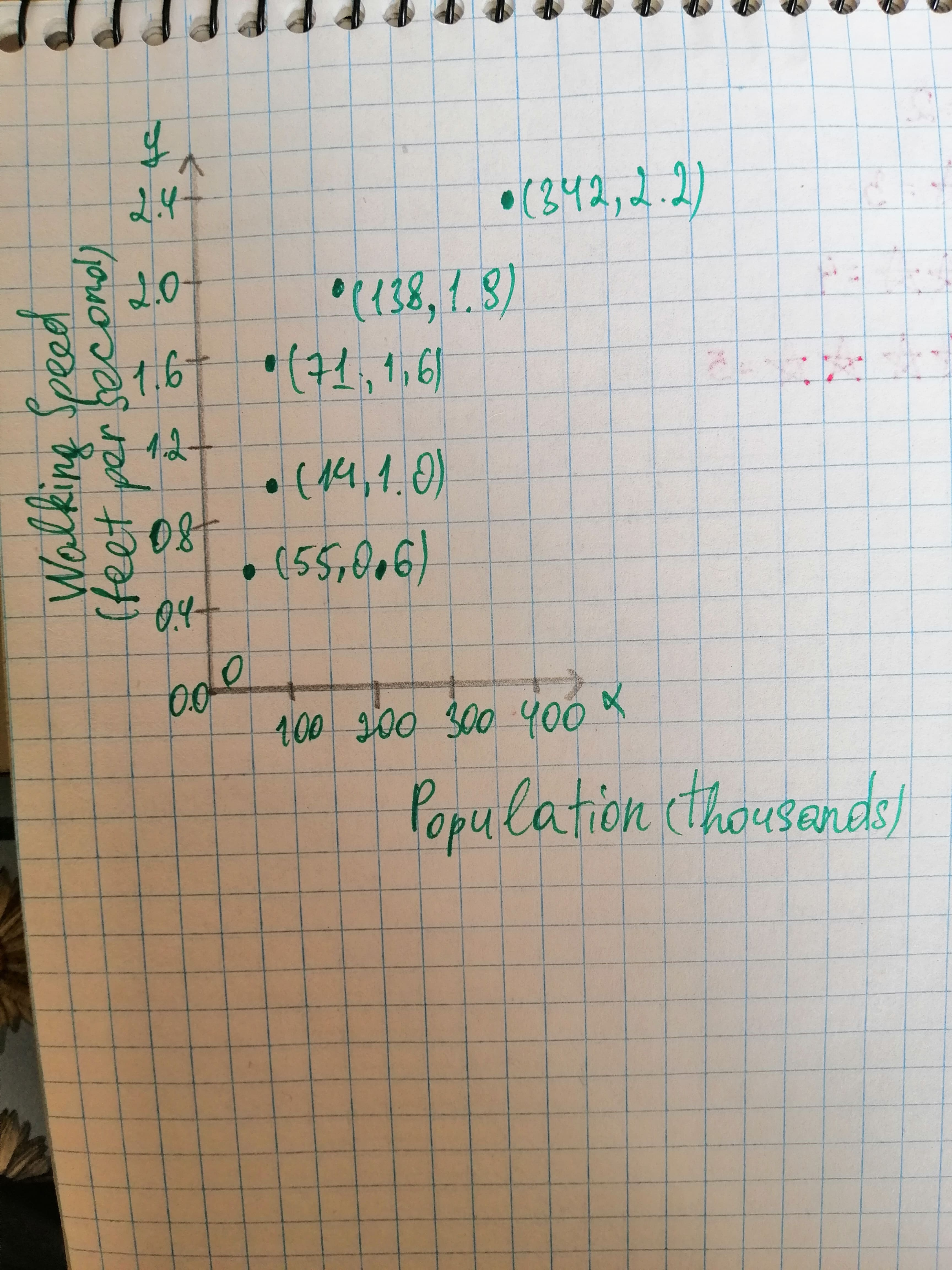Chardonnay Felix

2020-12-28

The table shows the population of various cities, in thousands, and the average walking speed, in feet per second, of a person living in the city.toroztatG

Graph: Consider Walking speed as y-coordinates and population as x-coordinate and the table for this is given below:

Use these points to plot the scatter plot. Thus, the scatter plot for the data is:Interpretation: The data in the scatter plot increase rapidly at first and then begin to level off a bit, the shape suggests that the logarithmic function is good choice for modeling the data.

Do you have a similar question?